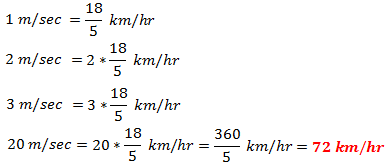1. Worksheets>
2. Math>
3. Solve>
4. Speed>
5. Conversion 2

# How to convert m/sec into km/hrTo convert m/sec into km/hr, multiply the number by 18 and then divide it by 5.

Need detail explanation? Go to bottom

Solved Example 1:

Convert 20 m/sec into km/hr.

Solution:

20 m/sec

Step 1:

Multiply 20 by 18

We have 20 * 18 = 360

Step 2:

Divide 360 by 5

360/5 = 72

20 m/sec = 72 km/hr

Try yourself: Practice Sheet 1

Solved Example 2:

Convert 36 m/sec into km/hr.

Solution:

36 m/sec

Step 1:

Multiply 36 by 18

We have 36 * 18 = 648

Step 2:

Divide 648 by 5

648/5 = 129.6

36 m/sec = 129.6 km/hr

Try yourself: Practice Sheet 2

Better Understanding:

The problem can be solved using an unitary method.

A shopkeeper sells each pencil for 5 cents. How much do 4 pencils cost? 4 * 5 cents= 20 cents, isn't it? What is the cost of 7 pencils? Yes, your guess is right. It is 7 * 5 cents = 35 cents.

Let us work out the problem in example 1 as it explained here.